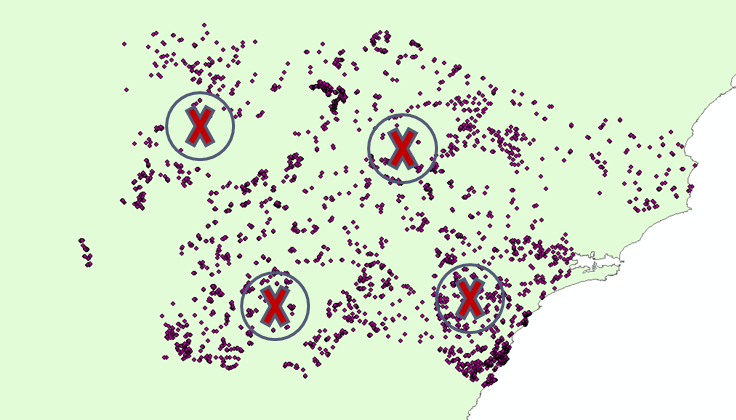## Tuesday, 29 April 2014

### Eco-Stats Lab April 2014: Block Bootstrap

In this weeks lab we learn about the block bootstrap. A non parametric way to deal with spatial auto correlation in your data and still make valid inferences.

### Bootstrap Recap

Bootstrapping allows us to find the unknown distribution of a statistic by resampling the original data (with replacement) and recalculating the statistic many times.
Hence we can calculate p-values and standard errors of things we don’t know the distribution of.
Assumptions: observations are independent and identically distributed ("iid")

### But you can't use an iid bootstrap when data are spatially correlated

Problem: When data points are spatial or temporal the independence assumption is (often) violated.

If ignored, this can give us false confidence, leading to standard errors being underestimated and p-values being overestimate

Solution: Resample in blocks that capture the correlation structure in the data. The bootstrap replicates of the statistic will reflect the correlation structure and give better answers.

Choose a block length "L". Then sample spatial points in the regions. For each point, collect the sites within a radius "L" of that point. Then paste all these sites together to form a new dataset on which you can calculate the test statistic.

### Diagram:### In R:

(Collect the file RLabBootstrap.RData from dropbox)

https://www.dropbox.com/s/tinsk65owlczhfy/RLabBootstrap.RData

#### #Prelude: read in the data

#load in a dataset and some functions. You might also need to..
#install.Packages(mvabund)

library(mvabund)

#### #Example 1

#Which of Winter minimum temps, (mint5), summer maximum temps (maxt95) and winter maximum temps (maxt5) have an effect on ferns in the family Blechnum?

#### #Bootstrap Recap

#Lets do a many glm anova using iid resampling in mvabund. Revision of stats course.

presence.Blechnum = mvabund(dat[,4:13])

model1 = manyglm(presence.Blechnum ~   poly(mint5,2) + poly(maxt95,2) + poly(maxt5,2) , data = dat, family = "binomial")
anova1 = anova(model1, nBoot=200) #nBoot=1000 is default, but we're saving time here. This step takes a minute

anova1 #they all appear to have a significant effect

#### #How to do a Block Bootstrap hypothesis test

#Resample blocks of length L using  resample_blocks(x=vector of x coordinated of sites, y=vector of y coordinates of sites, NBoot=number of bootstraps, block_L= block length).

BlockResampledSamples = resample_blocks(x, y, NBoot = 200, block_L = 20000) #Here I chose a block length of 20km and 200 bootstraps replicates

anova2 = anova(model1, bootID=t(BlockResampledSamples)) #runs a block bootstrap with 200 bootstrap reps and block length of 20km

#### #Coding: make it faster for repeat applications

# Creating a look up table makes resampling faster, on repeat resamples. E.g. if you decided to change nBoot. This step is especially important, computationally, the larger your region and the smaller the block length you want to try

lookup_table_10k = create_moving_block_lookup_RLAB(x,y,block_L = 10000, scale1 = 3000)

BlockResampledSamples.10k = resample_blocks(x, y, NBoot = 200,  lookup_table = lookup_table_10k) #Here I chose a block length of 10km by using the 10km lookup table

#### #What about L? Doesn't that affect my results?

#try 10km

anova3 = anova(model1, bootID=t(BlockResampledSamples.10k)) #you should use nBoot=1000 if you have

#In theory as block length L increases the estimate of the standard error will increase (tangentially). The bias of the standard error decreases as L increases but the variance increases. You could try a range of values of L and look for where the increase in the standard error seems to be tapering off. You don't want L to be too large as then you might be introducing unwanted variability into your answer. In this example using a block length of 1km (not shown) results in a similar p-value to a block length of 20km suggesting most of the correlation is within ~1km range. Thus it would be better to use a block length of closer to 1km than 20km.

### #(Probably homework) Using a Block bootstrap to calculate standard errors of ``things''.

#Example 2. What is the effect of mint5 and maxt95 on the probability of finding Blechnum.patersonii? Find the standard error of the effect size.

#first code a function to find the term "T" you want the standard error of.

Extract.Coeff.Maxt95=function(dat){
fit=glm(Blechnum.cartilagineum~mint5+maxt95,data=dat,family="binomial")
fit\$coeff
}

#then use bootstrap_wrapper() to calculate the term "T" many times on new datasets.

T_reps = bootstrap_wrapper(dat = dat, function_to_repeat =Extract.Coeff.Maxt95 , new_sample = BlockResampledSamples )

#Then calculate the standard deviation of the bootstrap replicates of the term T.

SE.Coeff_blocked=apply(data.frame(T_reps),1,sd)

#The standard errors are:
SE.Coeff_blocked

### #Also worth knowing:

#Time series block bootstrap using tsboot() in "boot" package.

library(boot)
data(lynx)
plot(log(lynx)) #a time series

#a function to calculate the order and mean of the series

lynx.fun <- function(tsb) {
ar.fit <- ar(tsb, order.max = 25)
c(ar.fit\$order, mean(tsb))
}

# the (temporal) block bootstrap with block length 20
lynx.1 <- tsboot(log(lynx), lynx.fun, R = 99, l = 20, sim = "fixed")

#block bootstrap SE/ bias of the "order" of the autoregressive time series and the mean of the time series
#order of autoregressive time series is the number of previous states it depends on

lynx.1

1.2.